Copyright © Michael Richmond. This work is licensed under a Creative Commons License.

# The shape of spectral lines

Our goal is to figure out the abundance of different chemical elements in a star from the appearance of its spectrum. The Boltzmann and Saha equations are part of the procedure, but there's another piece to to the puzzle: how to translate the width and/or depth of a spectral line into the number of atoms of material. That's the topic for today and tomorrow.

#### Weak lines and strong lines

Let's look at a portion of the solar spectrum in the red, from 6500 to 6600 Angstroms. There are many fewer lines here than in the blue portion of the spectrum, so it's easier to see details of individual features.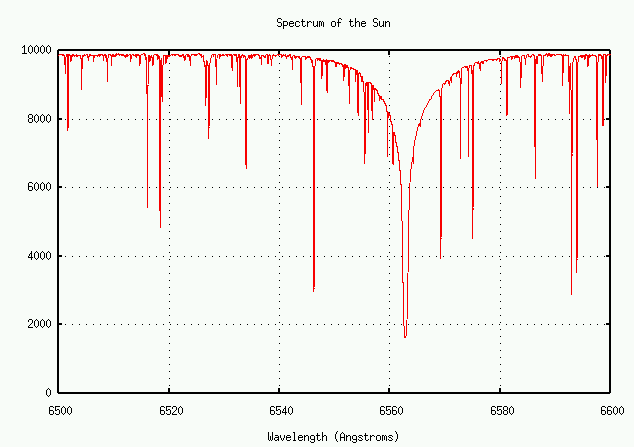There's a big whopping line in this region. What is it?

Exercise:
1. What element, and what transition, creates this line?
2. What is the equivalent width of the strong line?

On the other hand, there are also many weaker lines. Let's look more closely at the one near 6546 Angstroms: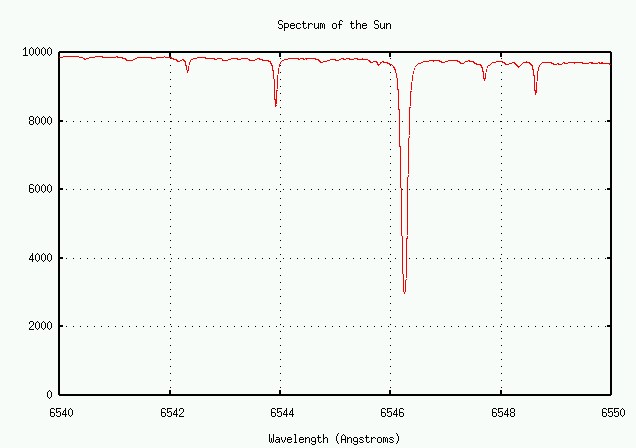Let's zoom in some more ...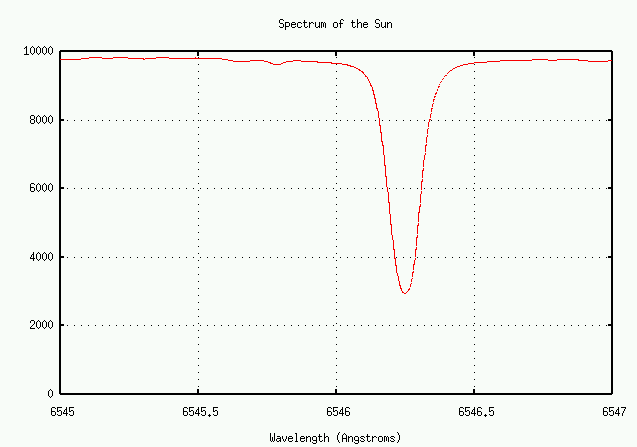Exercise:
1. What is the equivalent width of this weak line?

Does the weak line have the same shape as the really, really strong one? Well, let's plot them both together and find out. I'll modify the horizontal scale of the weaker line so that it fits into the same graph as the strong one.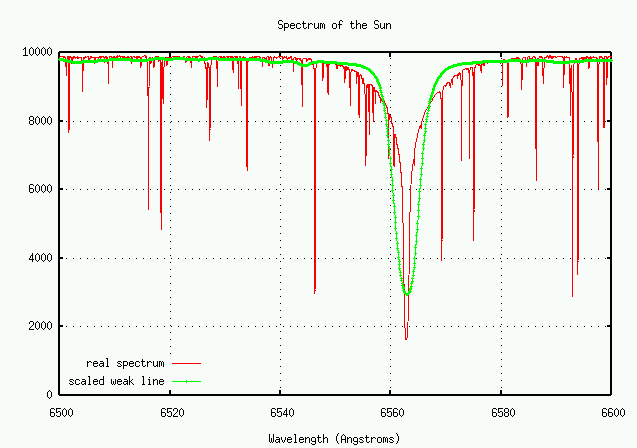No! The weak line appears to have a wider core, but narrower wings. Why is that?

Atoms emit (or absorb) light when their electrons jump from one energy state to another. In the case of the H-alpha line, the transition involves the n=3 and n=2 states of hydrogen.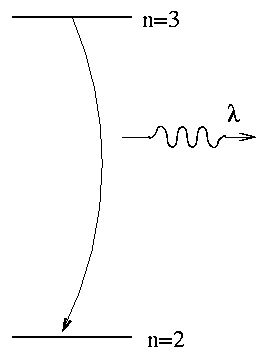If we knew the energy difference exactly, then we would know the wavelength of the photon exactly. However, due to Heisenberg's Uncertainty Principle, we do NOT know the energies exactly. One way to look at is that atoms very quickly jump from an excited state to a lower state; that means that you don't have very long to measure the energy in the upper state. The limit on the time available to measure the excited state's energy places a limit on the precision of the energy measurement:The lifetime of hydrogen atoms in their excited states is very short. For example, the lifetime of hydrogen in the n=2 and n=3 states is about 10^(-8) seconds.

Exercise:
1. Estimate the uncertainty in the energy of a Balmer-alpha photon.
2. Estimate the uncertainty in the wavelength of a Balmer-alpha photon.
3. Compare this to the equivalent widths of the Balmer-alpha line and the weak line in the actual solar spectrum.

If the atoms emitting and absorbing light are moving towards and/or away from an observer, then he will see Doppler shifts in the wavelengths: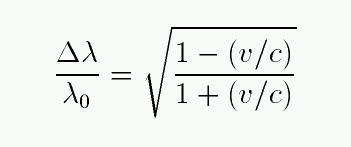where the velocity v is taken to be positive for motion AWAY from the observer, and negative for motion towards the observer. For the common case of non-relativistic motion, this simplifies to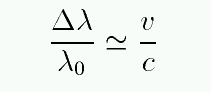Exercise:
1. Estimate the speed of hydrogen atoms in the photosphere where T = 6000 K.
2. Calculate the typical size of the Doppler shift due to random atomic motion.
3. Compare this to the equivalent widths of the Balmer-alpha line and the weak line in the actual solar spectrum.

Some stars suffer from turbulence: large-scale, coherent flows of material in bulk. If the star is cool (so thermal motions are relatively slow), and the turbulence is sufficiently strong, the Doppler shift due to bulk motion may exceed that of molecular motion.

How important is this on the Sun? Let's see .... take a look at this movie of the Sun, which comes from a collection of solar movies taken by the Dutch Open Telescope.

This movie shows a region of the solar photosphere 52 by 35 arcseconds across. Note the clock in the lower-left corner.

Exercise:
1. How long does the movie last?
2. How long does a typical cell last?
3. How wide is a typical cell, in km?
4. Assume that a granulation cell is thin: about 1/10 as high as it is wide. Assume that the gas makes one complete cycle during the life of a cell. What is the bulk velocity of the gas?
5. How does this compare to the random velocity of individual H atoms in the gas?

Atoms in a cloud of gas experience two types of perturbations from their neighbors. They collide directly with some, and feel the electric fields of the electrons passing close by; these two effects lead to collisional and pressure broadening, respectively.

To a rough approximation, we can estimate the collisional effect with the uncertainty principle. The typical time between collisions limits our ability to measure the exact energy of an atom, because its state will change with each collision. So, just how long do we have between collisions?

Exercise:
1. What is the mean free path of atoms in the photosphere of the Sun?
2. What is the typical speed of hydrogen atoms in the photosphere?
3. Calculate a typical time between collisions.
4. Use this time and Heisenberg's Uncertainty Principle to estimate the uncertainty in the energy of an atom in its excited state.
5. Estimate the uncertainty in the wavelength of a Balmer alpha photon which results from collisional broadening.
6. Compare the size of collisional broadening in the Sun to the other two causes of line width.Copyright © Michael Richmond. This work is licensed under a Creative Commons License.